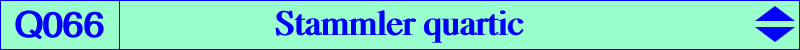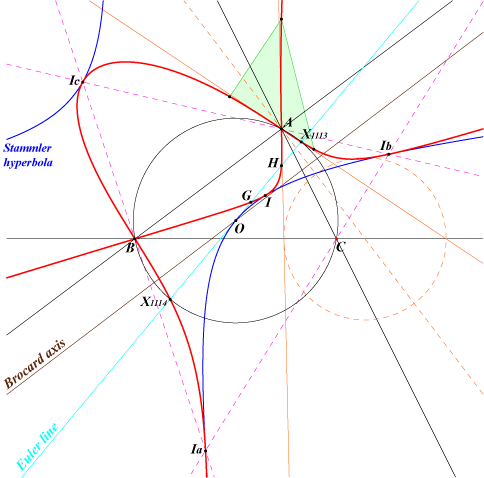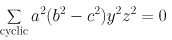X(1), X(2), X(4), X(254), X(1113), X(1114), X(1138), X(2184), X(3223), X(3346), X(3459) excenters, vertices of the antimedial triangle more generally, vertices of the anticevian triangle of any of its points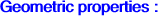Q066 is the isogonal transform of the Stammler rectangular hyperbola. This is the diagonal hyperbola passing through X(1), X(3), X(6), X(155), X(159), X(195), X(399), X(610), X(1498), X(1740), X(2574), X(2575), X(2916), X(2917), X(2918), X(2929), X(2930), X(2931), X(2935), X(2948) and the vertices of the anticevian triangle of any of its points. Q066 is also the square root of the circum-conic passing through X(2), X(6), X(25), X(37), X(42), X(111), X(251), X(263), X(308), X(393), X(493), X(494), X(588), X(589), X(694), X(941), X(967), X(1169), X(1171), X(1218), X(1239), X(1241), X(1383), X(1400), X(1427), X(1880), X(1976), X(1989), X(2054), X(2165), X(2248), X(2350), X(2395), X(2433), X(2963), X(2981), X(2987), X(2998). In other words, Q066 contains the square roots of all the points on the conic. Q066 is also the cyclocevian transform of the Kiepert hyperbola i.e. the cyclocevian conjugate M' of a point M on the Kiepert hyperbola ends up on Q066. Here is a list of such pairs {M,M'} : {2,4}, {4,2}, {13,13483}, {14,13484}, {1029,1}, {6504,3346}, {6625,8049}, {13576,9510}. (Peter Moses, 2017-06-15). See property 4 below. The points A, B, C are nodes. The nodal tangents pass through the traces of the circles orthogonal to the circum-circle and centered at the intersections of the perpendiculars at A, B, C to the Brocard axis with the sidelines of ABC. Q066 is the locus of point P such that the cevian and pedal triangles of P are orthologic the cevian and reflection triangles of P are orthologic. See Table 7. Other locus properties : Let P be a point and PaPbPc its cevian triangle, HaHbHc the orthic triangle (cevian triangle of H). The parallel through the point Pb to the sideline HaHb and the parallel through the point Pc to the sideline HaHc intersect at A'. The points B' and C' are defined likewise. The triangles ABC and A'B'C' are perspective if and nly if P lies on the Stammler quartic together with the circumcircle (Angel Montesdeoca, Hyacinthos #21817). Let Q be a fixed point. The locus of P such that the line QP*(P* = isogonal of P) is perpendicular to the trilinear polar of P is a quartic C(Q). When Q = O, the quartic is Q066. (Angel Montesdeoca, private message 2013-11-30). See also http://amontes.webs.ull.es/otrashtm/HechosGeometricos.htm#HG091114. The locus of P whose cevian circle passes through X(115) is Q066, together with the Kiepert hyperbola. (Angel Montesdeoca, private message 2016-01-27). See a generalization below. See also here (Angel Montesdeoca, 2018-04-17, in Spanish) and here (Angel Montesdeoca, 2019-12-03, in Spanish). Let A1B1C1 and A2B2C2 be the cevian and anticevian triangles of P whose trilinear polar (perspectrix of the triangles) meets the sidelines of ABC at A3, B3, C3. The circles AA1A3, BB1B3, CC1C3 are coaxial if and only if P lies on Q066. In this case, they meet at two points that lie on a line passing through X(6) and on K018. The midpoint of these two points lies on K1275 where other properties are mentioned. (This answers a question raised by Dan Reznik, 2023-02-10). Note that the circles AA2A3, BB2B3, CC2C3 are coaxial if and only if P lies on a (not very interesting) circum-septic passing through G, the in/excenters, the midpoints of ABC.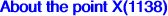X(1138) is the isogonal conjugate of X(399), the Parry reflection point. These two points lie on the Neuberg cubic. Jean-Pierre Ehrmann has shown that, except the trivial case H, X(1138) is the only point such that the (blue) pedal and (orange) cevian triangles are (indirectly) similar. These triangles are also simultaneously orthologic and parallelogic. See Table 7 and Table 8.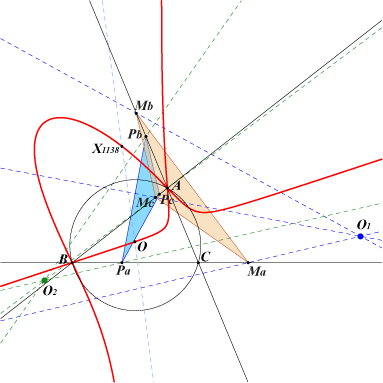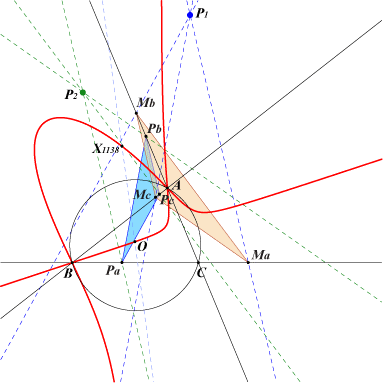Orthologic pedal and cevian triangles of X(1138) with centers of orthology O1 and O2 Parallelogic pedal and cevian triangles of X(1138) with centers of parallelogy P1 and P2*** The same facts remain true with the cevian and reflection triangles of X(1138) : these two triangles are simultaneously (indirectly) similar, orthologic and parallelogic. One of the centers of orthology (resp. parallelogy) is O1 (resp. P1).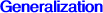(contributed by Angel Montesdeoca, 2016-01-27) Let F be a finite point. The locus of P whose cevian circle passes through F is generally a sextic S(F) and the isogonal transform of S(F) is a cubic K(F). The discriminant of K(F) vanishes (i.e. K(F) has at least one double point) if and only if : • F lies on one in/excircle in which case K(F) is in general a nodal cubic with node X(55) or extraversion. • F lies on the nine point circle in which case K(F) splits into a line passing through X(3) and a circum-conic passing through X(6). Hence S(F) is the union of a rectangular circum-hyperbola H(F) with center F and a circum-quartic Q(F) passing through X(2) with nodes at A, B, C. When F = X(115), Q(F) is Q066 and H(F) is the Kiepert hyperbola as said above. When F = X(125), Q(F) is Q124 and H(F) is the Jerabek hyperbola. Note that Q066 is the barycentric product of Q124 by X(1). See here for further examples. See also Q182, a similar quartic, the barycentric product of Q124 by X(6).